# Forecasting Process Details

### Predictions and Prediction Errors

Predictions are made based on the last known smoothing state. Predictions made at timeforsteps ahead are denoted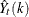and the associated prediction errors are denoted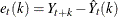. The prediction equation for each smoothing model is listed in the following sections.

The one-step-ahead predictions refer to predictions made at time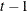for one time unit into the future—that is,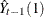. The one-step-ahead prediction errors are more simply denoted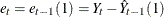. The one-step-ahead prediction errors are also the model residuals, and the sum of squares of the one-step-ahead prediction errors is the objective function used in smoothing weight optimization.

The variance of the prediction errors are used to calculate the confidence limits (Sweet, 1985; McKenzie, 1986; Yar and Chatfield, 1990; Chatfield and Yar, 1991). The equations for the variance of the prediction errors for each smoothing model are listed in the following sections.

Note: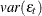is estimated by the mean square of the one-step-ahead prediction errors.